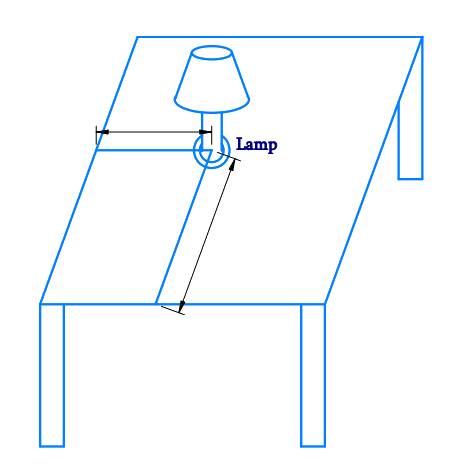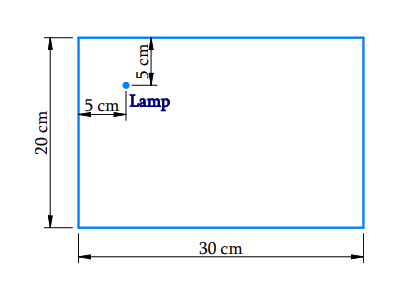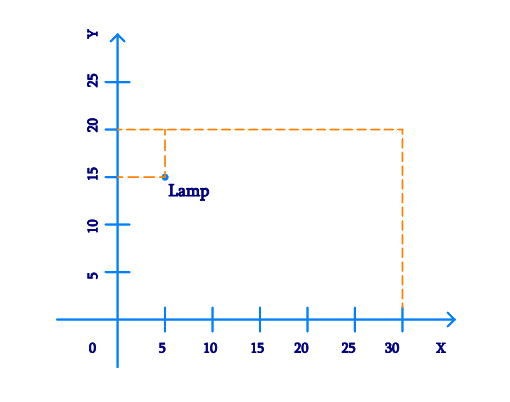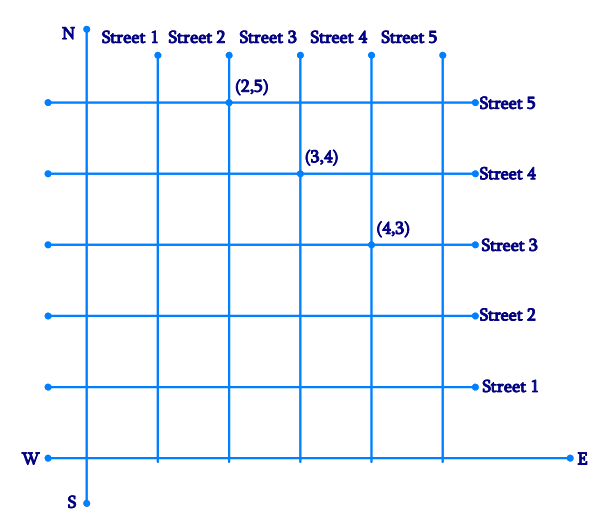# Exercise 3.1 Coordinate Geometry NCERT Solutions Class 9

## Chapter 3 Ex.3.1 Question 1

How will you describe the position of a table lamp on your study table to another person?

### Solution

Steps:The above figure is a $$\rm3-D$$ shows a study table, on which a study lamp is placed.

Now let us consider only the top surface of the table which becomes a $$\rm2-D$$ rectangle figure.From the Figure Above,

• Consider the lamp on the table as a point
• Consider the table as a $$\rm2-D$$ plane.

The table has a shorter side ($$20\,\rm cm$$) and a longer side ($$30\,\rm cm$$).

• Let us measure the distance of the lamp from the shorter side and the longer side.
• Let us assume
• Distance of the lamp from the shorter side is $$5 \rm\;{cm}.$$
• Distance of the lamp from the longer side is $$5 \rm\;{cm}.$$Therefore, we can conclude that the position of the lamp on the table can be described with respect to the order of the axes as ($$5,15$$).

## Chapter 3 Ex.3.1 Question 2

(Street Plan): A city has two main roads which cross each other at the center of the city. These two roads are along the North–South direction and East–West direction.

All the other streets of the city run parallel to these roads and are $$200\,\rm{ m}$$ apart. There are $$5$$ streets in each direction. Using $$1\, \rm{cm}$$ $$=$$ $$200\,\rm{ m}$$, draw a model of the city on your notebook. Represent the roads/streets by single lines. There are many cross- streets in your model.

A particular cross-street is made by two streets, one running in the North–South direction and another in the East– West direction. Each cross street is referred to in the following manner: If the $$2\rm{nd}$$ street running in the North–South direction and $$5$$th in the East–West direction meet at some crossing, then we will call this cross-street ($$2, 5$$). Using this convention, find:

(i) How many cross - streets can be referred to as ($$4, 3$$).

(ii) How many cross - streets can be referred to as ($$3, 4$$).

### Solution

Steps:

Let us draw two perpendicular lines as the two main roads of the city that cross each other at the center. Let us mark them as North–South and East–West.

As given in the question, let us take the scale as $$1\; \rm{cm} =$$ $$200\,\rm{ m}$$

Draw five streets that are parallel to both the main roads (which intersect), to get the given below figure.

Street plan is as shown in the figure:(i) There is only one cross street, which can be referred as ($$4, 3$$).

(ii) There is only one cross street, which can be referred as ($$3, 4$$).

Instant doubt clearing with Cuemath Advanced Math Program